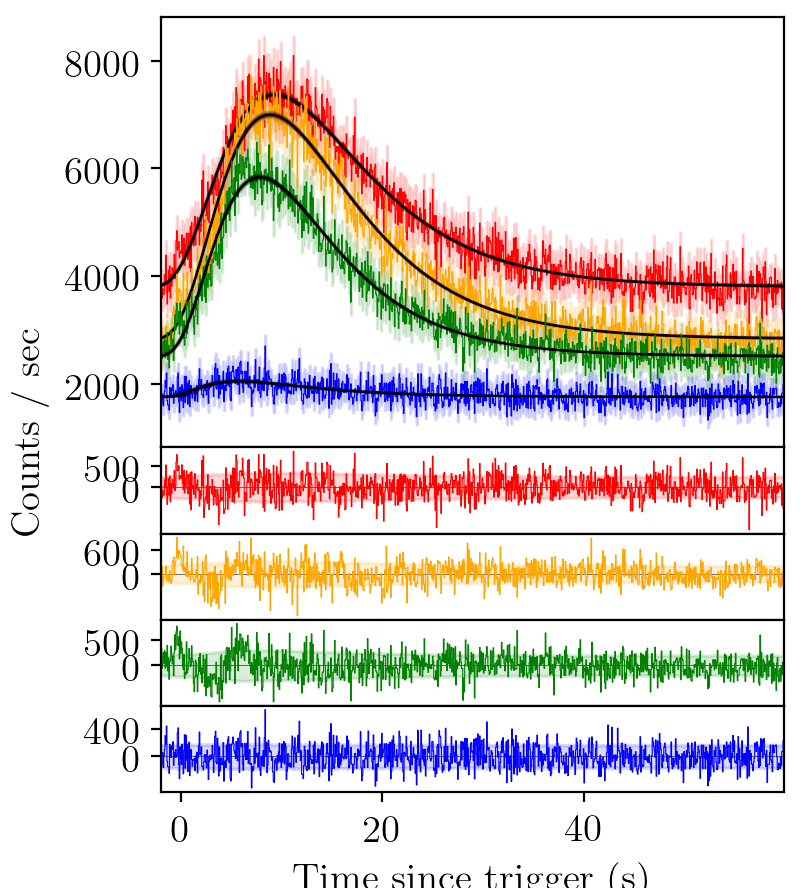# Pulse Types¶

A standard gamma-ray burst looks like this

## Gaussian pulse¶

A simple pulse parameterisation one might imagine is a Gaussian, like those used to model emission lines in eg. quasar spectra. The equation for a Gaussian pulse is:

$S(t|A,\Delta,\sigma) = A \exp \left[ \frac{\left( t - \Delta \right)^2}{2\sigma^2} \right]$

However, we can immediately see that such a pulse parameterisation does not catch the fast rise of the GRB pulse, nor the slow decay. The residuals have consistent structure.

## FRED pulse¶

The standard pulse parameterisation used to model gamma-ray bursts is a fast-rise exponential-decay (FRED) curve.

$S(t|A,\Delta,\tau,\xi) = A \exp \left[ - \xi \left( \frac{t - \Delta}{\tau} + \frac{\tau}{t-\Delta} \right) \right]$

The fit is better than a Gaussian, but again there is structure un-accounted for in the residuals.

## FRED-X pulse¶

We try again with an extended fast-rise exponential-decay model, FRED-X.

$S(t|A,\Delta,\tau,\xi,\gamma,\nu) = A \exp \left[ -\xi^\gamma \left(\frac{t - \Delta}{\tau}\right)^\gamma - \xi^\nu \left(\frac{\tau}{t-\Delta}\right)^\nu\right]$

Again, an element of structure in the residual persists.

## Sine-Gaussian residual¶

We use a sine-gaussian residual function to account for these residuals.

$\text{res}(t)= A_\text{res} \exp \left[ - \left(\frac{t-\Delta_\text{res}} {\lambda_\text{res}}\right)^2 \right] \cos\left(\omega t + \varphi \right)$BATSE trigger 7475 with FRED fit and sine-Gaussian residual (not implemented yet)

## A model selection script¶

Say, then, that we would like to fit on of each of these models in turn to the light-curve. To create a model, we specify a list of keys. For a Gaussian, FRED, and FRED-X pulse, the keys are as follows:

keys = ['G', 'F', 'X']


Residuals are included by placing a lower case ‘s’ following the pulse to which it should be applied. Residuals must be applied following a pulse, and cannot be used as a standalone. Let’s add an additional three pulse fits, all with a residual, to our list of keys.

keys += ['Gs', 'Fs', 'Xs']


So our complete list would look like

keys = ['G', 'F', 'X', 'Gs', 'Fs', 'Xs']


Now we need to convert our keys into models for the nested sampling analysis.

model_dict = {}
for key in keys:
model_dict[key] = create_model_from_key(key)
models = [model for key, model in model_dict.items()]


Finally, we feed each of the models in turn to the sampler through main_multi_channel. This function further splits the model (in this case into 4), and tests each of the channels individually.

for model in models:
GRB.main_multi_channel(channels = [0, 1, 2, 3], model = model)


The complete script for the above tutorial is here:

intermediate.py
from PyGRB.main.fitpulse import PulseFitter
from PyGRB.backend.makemodels import create_model_from_key

GRB = PulseFitter(7475, times = (-2, 60),
datatype = 'discsc', nSamples = 200, sampler = 'nestle',
priors_pulse_start = -5, priors_pulse_end = 30,
p_type = 'docs', HPC = False, )

keys = ['G', 'F', 'X', 'Gs', 'Fs', 'Xs']
# the last three models will take a non-trivial time to run
model_dict = {}
for key in keys:
model_dict[key] = create_model_from_key(key)
models = [model for key, model in model_dict.items()]

for model in models:
GRB.main_multi_channel(channels = [0, 1, 2, 3], model = model)
GRB.get_evidence_from_models(model_dict = model_dict)


The model selection script will output a set of tables that looks something like the following (residual models not included here for computational brevity).

Channel 1

 Model ln Z error ln BF G -4610.80 0.34 0.00 F -4181.16 0.38 429.64 X -4175.97 0.39 434.83

Channel 2

 Model ln Z error ln BF G -4912.74 0.34 0.00 F -4173.26 0.38 739.48 X -4164.73 0.39 748.01

Channel 3

 Model ln Z error ln BF G -4551.10 0.34 0.00 F -4043.61 0.37 507.49 X -4036.39 0.39 514.71

Channel 4

 Model ln Z error ln BF G -3714.97 0.28 0.00 F -3709.29 0.31 5.68 X -3709.44 0.31 5.53

Which tells us that a FRED-X model is preferred in this case for all channels.